## find the indefinite integral ∫((x^2)/(4x^3+9)) dx

Question

find the indefinite integral ∫((x^2)/(4x^3+9)) dx

in progress 0
2 months 2021-08-01T05:07:43+00:00 1 Answers 2 views 0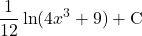Step-by-step explanation:

we would like to integrate the following indefinite integral: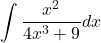in order to integrate it we can consider using u-substitution also known as the reverse chain rule and integration by substitution as well

we know that we can use u-substitution if the integral is in the following form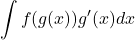since our Integral is very close to the form we can use it

let our u and du be 4x³+9 and 12x²dx so that we can transform the Integral

as we don’t have 12x² we need a little bit rearrangement

multiply both Integral and integrand by 1/12 and 12: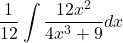apply substitution: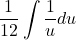recall Integration rule: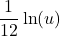back-substitute: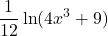finally we of course have to add constant of integration:and we are done!